MORE IN Basic Electrical & Electronics Engg
MU First Year Engineering (Semester 1)
Basic Electrical & Electronics Engg
December 2012
Total marks: --
Total time: --
INSTRUCTIONS
(1) Assume appropriate data and state your reasons
(2) Marks are given to the right of every question
(3) Draw neat diagrams wherever necessary

1(a) Find the current flowing through 5Ω resistance using source transformation.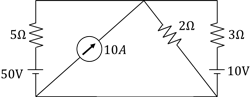3 M
1(b) State and explain maximum power transfer theorem
3 M
1(c) An alternating voltage is given by V = 141.4 sin 314t. Find
(i) Frequency
(ii) RMS Values
(iii) Average Value
(iv) Instantaneous value of voltage at t = 3msec.
3 M
1(d) Define the equation for resonance frequency (fr) in parallel resonance circuit.
2 M
1(e) Write down voltage, current and power relation in balanced delta connected load.
3 M
1(f) Derive emf equation for single phase transformer.
4 M
1(g) Draw complete V-I characteristics of a Diode
2 M

2(a) Determine the value of Resistance R as shown in figure using KVL and KCL.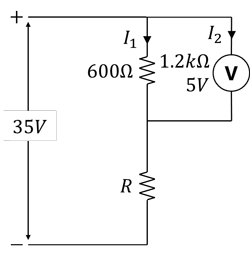6 M
2(b) A 100Ωresistor is connected in series with a choke coil. When a 400 V, 50Hz Supply is applied to this combination, the voltage across the resistance and the choke coil are 200 V and 300 V respectively. Find the power consumed by the choke coil. Also calculate the power factor of choke coil and power factor of the circuit.
8 M
2(c) Draw Phasor Diagram of single phase transformer on resistive load (unity power factor) and inductive load (lagging power factor).
6 M

3(a) Three similar coils, connected in star, take a total power of 1.5 kW at a p.f. of 0.2 lagging from a three phase, 440 V, 50 Hz Supply. Calculate the resistance and inductance of each coil.
8 M
3(b) A 230/110 V, single phase transformer takes an input of 350 VA at no load and at rated voltage. The core loss is 110W. Find (i) The iron loss component of no load current (ii) magnetizing component of no load current and (iii) No load power factor
6 M
3(c) Define filter and write down types of filters
2 M
3(d) Explain input characteristics of common emitter configuration.
4 M

4(a) Calculate Rxy for the circuit shown in Figure: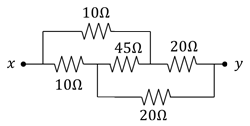7 M
4(b) A voltage of 150V, 50Hz is applied to a coil of negligible resistance and inductance 0.2H. Write the time equation for voltage and current.
5 M
4(c) In a balanced three phase circuit, power is measured by two wattmeters, the ratio of two wattmeter readings is 2:1. Determine the power factor of the system.
4 M
4(d) Explain with circuit diagram and waveform working of center tap full wave rectifier.
4 M

5(a) Determine the value of R for maximum power transfer. Also find the magnitude maximum power transferred.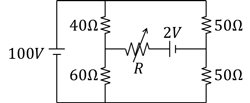8 M
5(b) Calculate the branch current I1 and I2 for the circuit shown below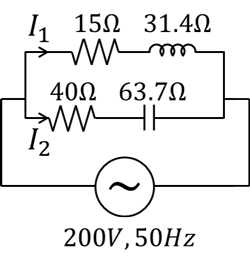4 M
5(c) A 30 kVA, 2400/120V, 50Hz transformer a high voltage winding resistance of 0.1Ω and a leakage resistance of 0.22Ω. The low voltage winding resistance is 0.035Ω and the leakage reactance is 0.012Ω. Calculate for the transformer
i. Equivalent resistance as referred to both primary and secondary.
ii. Equivalent reactance as referred to both primary and secondary.
iii. Equivalent impedance as referred to both primary and secondary.
iv. Copper loss at full load.
8 M

6(a) Find the current through 3Ω resistor using superposition theorem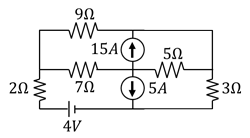7 M
6(b) A resistor and a capacitor are connected in series with a variable inductor. When the circuit is connected to a 230 V, 50 Hz supply, the maximum current obtained by varying the inductance is 2A. The voltage across the capacitor is 500 V. Calculate the resistance, inductor and capacitor of the circuit.
7 M
6(c) Explain measurement of three phase power using two wattmeter method.
6 M

More question papers from Basic Electrical & Electronics Engg